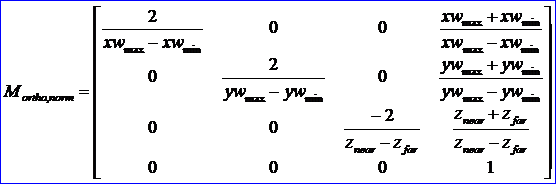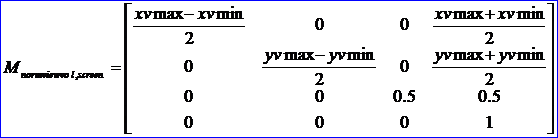0
0
01. 云栖社区>
2. 博客>
3. 正文

eryar 2014-12-07 17:01:00 浏览766

Abstract. The purpose of the OpenGL graphics processing pipeline is to convert 3D descriptions of objects into a 2D image that can be displayed. In many ways, this process is similar to using a camera to convert a real-world scene into a 2D print. To accomplish the transformation from 3D to 2D, OpenGL defines several coordinate spaces and transformations between those spaces. Each coordinate space has some properties that make it useful for some part of the rendering process. The transformations defined by OpenGL afford applications a great deal of flexiblity in defining 3D-to-2D mapping. So understanding the various transformations and coordinate spaces used by OpenGL is essential. The blog use GLUT to demenstrate the conversion between 3D and 2D point.

Key Words. OpenCASCADE, OpenGL, Coordinate Transform,

1. Introduction

2.From 3D to 2D

v 模型视点变换MODELVIEW TRANSFORMATION；

v 投影变换PROJECTION TRANSFORMATION；

v 视口变换VIEWPORT TRANSFORMATION；

Figure 2.1 OpenGL Vertex Transformation Pipeline

Figure 2.2 Stages of Vertex Transformation(OpenGL Programming Guide)

Figure 2.3 The vertex transformation pipeline(OpenGL SuperBible)

2.1 模型视点变换Model-View Transformation

Figure 2.4 From World Cooridante System to Viewing Coordinate System

2.2 投影变换Projection Transformation

Figure 2.5 Orthographic Viewing Volume(OpenGL Programming Guide)

Figure 2.6 Orthogonal Projection View Volume to Normalized View Volume棱台形状的视景体又称为视锥体，主要用于完成透视投影的工作，这种投影更符合人们的心理习惯，即离视点近的物体较大，离视点远的物体较小。在OpenGL中由函数glFrustum和gluPerspective来实现。

Figure 2.7 Perspective Viewing Volume Specifiedc by glFrustum

2.3 视口变换 Viewport Transformation

Figure 2.8 Mapping the Viewing Volume to the Viewport综上所述，场景从三维世界坐标系变换到屏幕窗口的所有变换过程进行叠加，则得到如下的变换公式：

3.From 2D to 3D

Figure 3.1 Viewport cooridnate to World Coordinate(By Zhangci)

Figure 3.2 OpenSceneGraph TranslateAxisDragger

4.Code Example

void BuildNorm2ViewportMatrix(GLsizei theWidth, GLsizei theHeight)
{

// row 0
theNorm2Viewport.ChangeValue(00= (theWidth - 0/ 2.0;
theNorm2Viewport.ChangeValue(
01= 0.0;
theNorm2Viewport.ChangeValue(
02= 0.0;
theNorm2Viewport.ChangeValue(
03= (0 + theWidth) / 2.0;

// row 1
theNorm2Viewport.ChangeValue(10= 0.0;
theNorm2Viewport.ChangeValue(
11= (0 - theHeight) / 2.0;
theNorm2Viewport.ChangeValue(
12= 0.0;
theNorm2Viewport.ChangeValue(
13= (theHeight + 0/ 2.0;

// row 2
theNorm2Viewport.ChangeValue(20= 0.0;
theNorm2Viewport.ChangeValue(
21= 0.0;
theNorm2Viewport.ChangeValue(
22= 0.5;
theNorm2Viewport.ChangeValue(
23= 0.5;

// row 3
theNorm2Viewport.ChangeValue(30= 0.0;
theNorm2Viewport.ChangeValue(
31= 0.0;
theNorm2Viewport.ChangeValue(
32= 0.0;
theNorm2Viewport.ChangeValue(
33= 1.0;
}

Figure 4.1 Test Coordinate Transformation

5.Conclusion

6. References

1. Dave Shreiner. OpenGL Programming Guide(7th Edition). Addison-Wesley. 2010

2. Richard S. Wright Jr., Benjamin Lipchak. OpenGL SuperBible. Sams. 2004

3. Randi J. Rost. OpenGL Shading Language(2nd Edition). Addison-Wesley. 2006

4. Donald Hearn, M. Pauline Baker. Computer Graphics with OpenGL. 2004

5. Zhangci. OpenGL Rendering Pipeline&Coordinate Transformation.

6. 王锐, 钱学雷. OpenSceneGraph三维渲染引擎设计与实践. 清华大学出版社. 2009